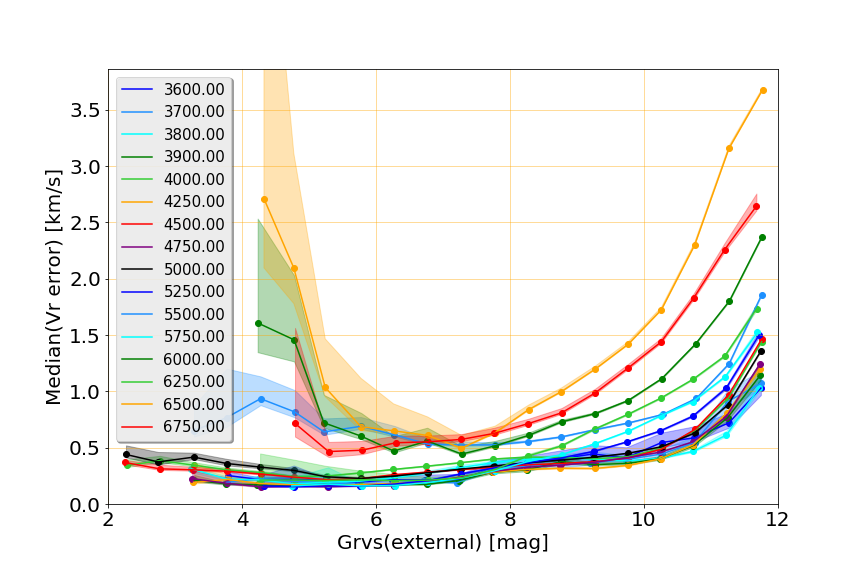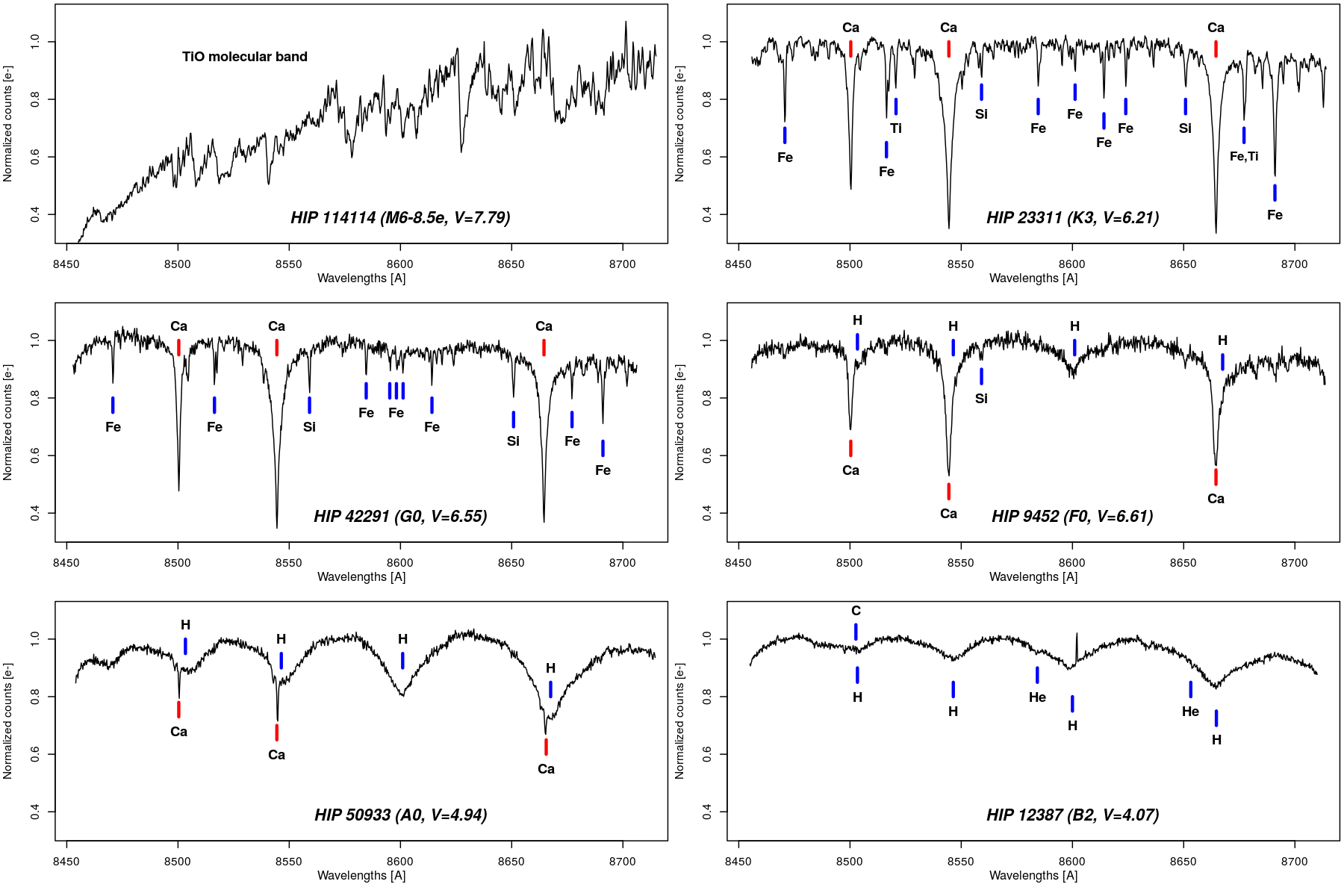# 6.5.2 Validation

When the processing was finished, the CU6 carried out a validation campaign off-line on all pipeline $V_{\rm rad}$ results in order to assess the properties of the entire dataset and to identify the bad quality data to exclude from the Gaia DR2 publications (Katz et al. 2018). The spectroscopic pipeline produced the median radial velocity of 9 816 603 stars from which 2 344 881 have been excluded due to bad quality identified after the internal validation. The radial velocities of the stars with the following properties have been excluded. The approximate percentage of the sources removed by each criterion is also indicated. Some of the sources satisfy more than one criterion, and the percentage depends on the order the filtering criteria are applied:

• $V_{\rm rad}$ outside the limit STA range ]-1000; 1000[  km s${}^{-1}$(only 70 stars satisfy this criterion);

• coordinate uncertainties larger than 100 mas: i.e. $\sqrt{\sigma_{\alpha}^{2}+\sigma_{\delta}^{2}}>$100 mas ($\sim$ 0.3 %);

• $V_{\rm rad}^{\rm t}$ flagged as ambiguous in all transits by STA ($\sim$ 20.5 %);

• large radial velocity uncertainty (radial_velocity_error) $\sigma_{V_{\rm rad}}\geq 20$  km s${}^{-1}$($\sim$ 49.4 %);

• ‘hot’ stars having the associated template rv_template_teff $T_{\rm eff}$ $\geq$ 7000 K ($\sim$ 19 %);

• ‘cool’ stars with rv_template_teff $T_{\rm eff}$ $\leq$ 3500 K ($\sim$ 5.5 %);

• detected emission lines (templates for emission-line stars are not available and the $V_{\rm rad}^{\rm t}$ estimation cannot be done properly) ($\sim$ 0.2 %);

• too faint flux measured in the window: internal $G_{\rm RVS}$ $>14$ ($\sim$ 0.08 %);

• suspected binaries detected as double-lined in $>$ 90 % of the transits ($\sim$ 2.3 %);

• 397 stars with $|V_{\rm rad}|\geq$ 500  km s${}^{-1}$(the spectra of the 627 stars found to have $|V_{\rm rad}|\geq$ 500  km s${}^{-1}$, after the above filters had been applied, have been visually inspected and the radial velocities obtained with damaged spectra have been excluded).

Some other data have been excluded by the validation campaign carried out by the CU9 (Section 1.2.2). In particular, some stars have been excluded because they have been found to be the duplication of other stars. In total, in Gaia DR2 there are radial velocities for $\sim 7.2$ million stars. They have radial_velocity_error $<$ 20  km s${}^{-1}$(the median value is $\sim 1$  km s${}^{-1}$). Their associated template $T_{\rm eff}$ is in the range: $3500<$ rv_template_teff $<7000$ K.

The accuracy of the $V_{\rm rad}$ measurements has been estimated by comparison with the external catalogues (Table 6.3) even if also the external catalogues can be affected by systematic zero-point shifts. Figure 6.8 shows the median radial velocity residuals ($\Delta V^{\rm res}=V_{\rm rad}-V_{\rm rad}^{\rm cat}$) as a function of the external $G_{\rm RVS}$. The RVS $V_{\rm rad}$ of the bright stars do not exhibit a significant offset with the APOGEE and the CU6GB $V_{\rm rad}^{\rm cat}$, but they show an offset with SIM and RAVE. For stars fainter than $G_{\rm RVS}$ $\sim 9$, the RVS $V_{\rm rad}$ exhibit an offset increasing with magnitude with the other catalogues, and reaching approximately 500  m s${}^{-1}$ at the faint end. The reason for this is not yet understood but may be attributed, at least in part, to Charge Transfer Inefficiency due to radiation damage. The pre-launch end-of-mission requirement on the systematic uncertainty was $\sim 300$  m s${}^{-1}$ (Cropper et al. 2018), and the 500  m s${}^{-1}$ shift for the stars at the faint end is acceptable for this first data release.Figure 6.8: Median of the radial velocity residuals as a function of the external GRVS magnitude. A minimum of 20 stars per bin is required to calculate the median of the radial velocity residuals. The shaded area represent the robust uncertainty and are limited by the 15.85t⁢h and 84.15t⁢h percentiles. Figure from Katz et al. (2018).

Figure 6.9 presents the Gaia DR2 precision as a function of the external $G_{\rm RVS}$ magnitude and of effective temperature of the templates associated to the stars, rv_template_teff (the different curves). In general the radial velocity precision improves as the effective temperature decreases. This is the direct consequence of the morphology of the spectra depending on the temperature (see Figure 6.10). However some nearby curves show the opposite behaviour: the stars processed with $T_{\rm eff}$ = 6750 K templates (red curve) have better precision than those processed with $T_{\rm eff}$ = 6500 K templates (orange curve). The reason is that temperatures of 6500 K are associated to the star by the module DetermineAP (Section 6.4.4) and are more affected by template mismatch than the stars with $T_{\rm eff}$ = 6750 K which comes from the ground-based parameters (Section 6.2.3). The pre-launch end-of-mission requirement on the precision was $\sim 1$  km s${}^{-1}$for the G2V stars with $G_{\rm RVS}$ $\lesssim 12$ (Cropper et al. 2018). The radial velocities obtained for $G_{\rm RVS}$ $<11.5$ in this first data release are already exceeding the end-of-mission requirement.Figure 6.9: Precision: median of the radial velocity uncertainties (ϵVrad) as a function of the external GRVS magnitude. Curves: Teff templates (rv_template_teff). Figure from Katz et al. (2018).Figure 6.10: RVS spectra of six Hipparcos stars with different spectral types. The coolest star (M6 type) spectrum is dominated by the molecular TiO band. These stars, with Teff < 3500 K, are excluded from Gaia DR2. The spectral types K3 and G0 are included in Gaia DR2, the spectra are dominated by the CaII lines, and as the Teff increases the weak neutral lines such as Fe, Si, Ti are less numerous and their contrast with respect to the continuum decreases, this results in less information to derive the radial velocity, and explains why the precision is better for the cooler stars. In the F0 star the H lines start to appear and template mismatch on log⁡g results in imprecise Vrad. Stars with Teff ≥7000 K are excluded from Gaia DR2. Figure by Olivier Marchal, image of the week https://www.cosmos.esa.int/web/gaia/iow_20141124.